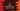# C program to find all Abundant numbers from 1 to 100## C program to find all Abundant numbers from 1 to 100:

In this post, we will learn how to find all abundant numbers from 1 to 100 in C programming language. Abundant numbers are also called Excessive numbers. It is a number which is smaller than the sum of its proper divisors.

If we need find all abundant numbers in a range, we have to write a function to check if a specific number is abundant or not. This function can be used with a loop to find all abundant numbers in a given range.

With this program, you will learn how to use functions in C, how to read user inputs and how to do mathematical calculations in C.

### Abundant number:

A number is called a abundant number or excessive number if the sum of its proper divisors is greater than the number itself.

12 is the first Abundant number because its proper divisors are 1, 2, 3, 4 and 6. The total is 16, which is larger than 12.

### Algorithm to find Abundant numbers from 1 to 100:

We will use the below algorithm in the C program:

• Run a loop from 1 to 100.
• Inside the loop, check for each number if it is an abundant number or not.
• To check if a number is abundant, we will use another loop.
• This loop will run from 1 to number - 1 and it will find all proper divisors of the number.
• If the sum of all proper divisors for a number is greater than the number, it is an abundant number.
• If an Abundant number is found, print it. Else, move to the next iteration of the loop.

### Method 1: C program to find abundant numbers from 1 to 100 using for loop:

Below is the complete C program to find all Abundant numbers from 1 to 100:

``````#include <stdio.h>

int isAbundant(int n)
{
int sum = 0;

for (int i = 1; i < n; i++)
{
if (n % i == 0)
{
sum += i;
}
}

return sum > n;
}

int main()
{
printf("Abundant numbers within 1 to 100 are: ");
for (int i = 1; i <= 100; i++)
{
if (isAbundant(i))
{
printf("%d ", i);
}
}
printf("\n");

return 0;
}``````

In this program:

• The main function runs first. It uses a for loop to find all abundant numbers from 1 to 100.
• Inside the loop, it calls the isAbundant function to check if the current value of i is an abundant number or not.
• If yes, it prints that number.
• The isAbundant function check if a specific number is an abundant number or not.
• The sum variable is initialized as 0 to hold the sum of all proper divisors.
• The for loop runs from i = 1 to i = n - 1. On each iteration, it checks if i is a proper divisor of the number or not.
• If yes, it adds the value of i to sum.
• Once the loop ends, it returns 1 if the sum is greater than the number. Else, it returns 0.
• If isAbundant returns 1, it prints that number.

#### Output:

If you run this program, it will print the below output:

``Abundant numbers within 1 to 100 are: 12 18 20 24 30 36 40 42 48 54 56 60 66 70 72 78 80 84 88 90 96 100 ``

### Method 2: C program to find abundant numbers from 1 to 100 using while loop:

We can also write the above program using while loops. Below is the complete program:

``````#include <stdio.h>

int isAbundant(int n)
{
int sum = 0, i = 1;

while (i < n)
{
if (n % i == 0)
{
sum += i;
}
i++;
}

return sum > n;
}

int main()
{
int i = 1;

printf("Abundant numbers within 1 to 100 are: ");

while (i <= 100)
{
if (isAbundant(i))
{
printf("%d ", i);
}
i++;
}
printf("\n");

return 0;
}``````

The only difference is that we are using while loops instead of for loops in this program. This program will given the same output.

``Abundant numbers within 1 to 100 are: 12 18 20 24 30 36 40 42 48 54 56 60 66 70 72 78 80 84 88 90 96 100 ``